home Home » Reasoning » Syllogims » Objective Questions

## Reasoning SyllogimsPage 2

###### Section-2
Direction: Three statements are given in each of the following questions, followed by three conclusions numbered 1, 2 and 3. You have to take the given statements to be true even if they seem to be at variance from commonly known facts. Read all the conclusions and then decide which of the given conclusions logically follows from the given statements, disregarding commonly known facts.

1.

 Statements: Some eye drops are ear drops. All eye drops are liquids. Some liquids are eye drinkable. Conclusions: 1. Some eye drops are drinkable. 2. Some ear drops are drinkable. 3. All liquids are eye drops. (a)1 and 2 follow (b)2 and 3 follow (c)All follow (d)None follows
Answer is: D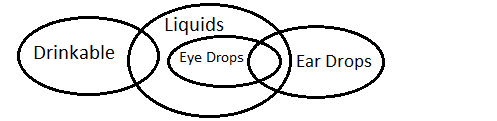Clearly, none of the given conclusions follows.

2.

 Statements: Nail polish is a cosmetic. No cosmetic is powder. Some powders are medicine. Conclusions 1. Some powders are cosmetics. 2. Some medicines are nail polishes. 3. Some cosmetics are nail polishes. (a)Only 1 follw (b)Only 2 follw (c)Only 3 follow (d)All follow
Answer is: C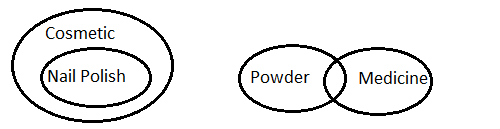Clearly, only some cosmetics are nail polishes.

3.

 Statements: All skaters are good swimmers. All good swimmers are runners. Conclusions: 1. Some runners are skaters. 2. Some skaters are good swimmers. (a)If only Conclusion 1 follows (b)If only Conclusion 2 follows (c)If either Conclusion 1 or 2 follow (d)If neither Conclusion 1 nor 2 follow
Answer is: C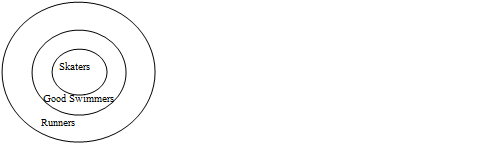Hence, Both Conclusions 1 and 2 follows.

4.

 Statements: Some buses are four wheelers. All four wheelers are vans. Conclusions: 1. Some vans are buses. 2. Some buses are vans. (a)If only Conclusion 1 follows (b)If only Conclusion 2 follows (c)If either Conclusion 1 or 2 follow (d)If neither Conclusion 1 nor 2 follow
Answer is: C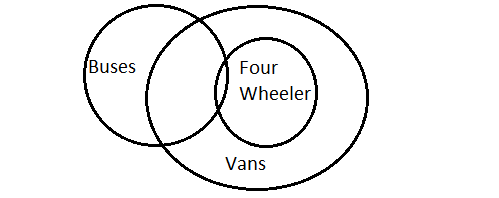Hence, Both conclusions 1 and 2 follow.

5.

 Statements: Some squares are circles. No circle is a triangle. No line is a square. Conclusions: 1. All squares can never be triangles. 2. Some lines are circles. (a)If only Conclusion 1 follows (b)If only Conclusion 2 follows (c)If either Conclusion 1 or 2 follow (d)If neither Conclusion 1 nor 2 follows
Answer is: A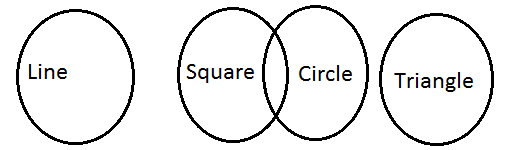From the figure we only can conclude that all squares can never be triangles.

6.

 Statements: Some squares are circles. No circle is a triangle. No line is a square. Conclusions: 1. No triangle is square. 2. No lines is a circle. (a)If only Conclusion 1 follows (b)If only Conclusion 2 follows (c)If either Conclusion 1 or 2 follow (d)If both Conclusions 1 and 2 follow
Answer is: DFrom the above figure, no triangle is a square and no line is a circles.

7.

 Statements: Some towers are window. All windows are houses. Some houses are temples. Conclusions: 1. Some towers are temples. 2. Some houses are temples. 3. Some temples are windows. (a)Only 1 follw (b)Only 2 follw (c)1 and 2 follow (d)2 and 3 follow
Answer is: B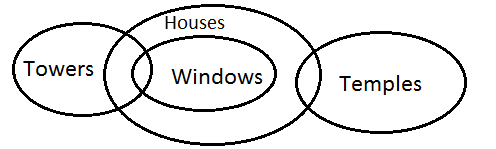Clearly, only Conclusion 2 follows.

8.

 Statements: Some walls are doors. Some doors are coats. Some coats are chairs. Conclusions: 1. Some chairs are doors. 2. Some coats are walls. 3. No chair is door. (a)Only 1 follw (b)Only 2 follw (c)Only either 1 or 3 follow (d)1 and 2 follow
Answer is: CClearly, only either 1 or 3 follows.

9.

 Statements: All dogs are rats. All rats are crows. All crows are parrots. Conclusion: 1. All dogs are parrots. 2. Some parrots are dogs. 3. Some crows are dogs. 4. All rats are dogs. (a)1 and 2 follow (b)1, 2 and 3 follow (c)Either 2 or 4 follow (d)Either 1 or 2 or 3 follows
Answer is: B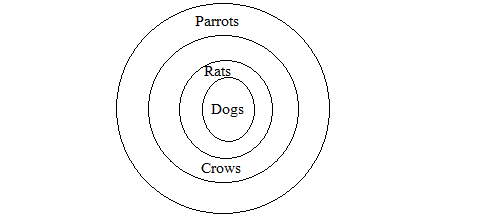Clearly, 1, 2 and 3 follows.

10.

 Statements: All rings are fingers. Some ears are fingers. All ears are necklaces. Conclusions: 1. Some necklaces are fingers. 2. Some necklaces are rings. (a)Only 1 follows (b)Only 2 follows (c)Both 1 and 2 follows (d)None follows
Answer is: A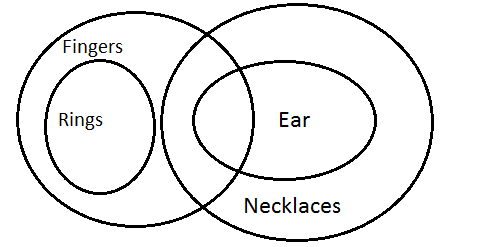Clearly, only Conclusion 1 follows.